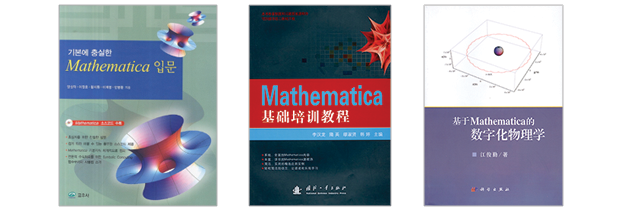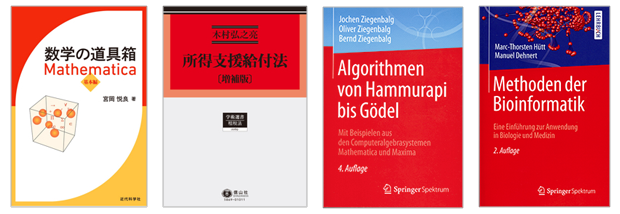Browse by Topic

# Wolfram Language Books around the World

The population of Wolfram Language speakers around the globe has only grown since the language’s inception almost thirty years ago, and we always enjoy discovering users and authors who share their passion for Wolfram technologies in their own languages. So in this post, we are highlighting foreign-language books around the world that utilize Wolfram technologies, from a mathematical toolbox in Japanese to an introduction on bioinformatics from Germany.## Basic Mathematica Primer (Korean)

Our first title is from a group of authors fluent in both Korean and the Wolfram Language. Introducing Korean-language speakers to the powerful fundamentals of Mathematica, the authors—Seong-Deog Yang, Jang hoon Lee, Jiwon Hwang, Chae Yeong Lee, and Myeong Hwan An—combine rich source code and friendly instructions for novices to the Wolfram Language, systematically covering all the basic knowledge needed to explore mathematics with Wolfram technology.

## Mathematica Basic Training Course (Chinese)

The Wolfram Language is widely used in China, with its tools for symbolic computation, graphics, high-precision calculations, and more. Hanlong Li, Ying Sui, Shuxian Miu, and Ting Han explore applications in higher mathematics, linear algebra, differential equations, probability, computing methods, operations research, and mathematical modeling with a large selection of examples, including advanced features in Mathematica 10. The authors even offer examples of writing and calling your own software packages to develop programs with the Wolfram Language.

## Mathematica-Based Digital Physics (Chinese)

Focusing on the domain of digital physics, Junqin Jiang combines Mathematica’s array of tools with research methods and programming design ideas to help the reader master physics concepts. With interactive examples covering quantum mechanics, electric and magnetic fields, and also numerical solutions and trajectories for dynamics and differential equations, Mathematica-Based Digital Physics promises to make learning the basic numerical methods for solving physics problems much easier than it would be with traditional textbooks.## Toolbox for Mathematics, Mathematica Basic (Japanese)

Etsuo Miyaoka has written a must-have book for not just Japanese Mathematica beginners but also for experienced users still exploring the features of Mathematica 10 and beyond. The first half is aimed at high-school- and early-college-level mathematics, and compares common mathematical expressions with Wolfram Language commands. The discussion then turns in the latter half to the mathematical expressions found in business and 3D schemes, and how Mathematica continues to be the definitive computing system to rely on.

## Income Support Benefits Law (Japanese)

One of the domains that the Wolfram Language continues to stand out in worldwide is finance and economics. In this augmented edition, Konosuke Kimura uses Mathematica to create not only the programs but also the accompanying graphics and visualizations for creating linear graduated tax tables, computing income gaps, and calculating income tax revenue and annual expenditures for income support benefits.

## Algorithmen von Hammurapi bis Gödel: Mit Beispielen aus den Computeralgebrasystemen Mathematica und Maxima, 4 Auflage (German)

Europe is also full of mathematicians, computer scientists, and other specialists that speak fluent Wolfram. Jochen Ziegenbalg, Oliver Ziegenbalg, and Bernd Ziegenbalg explore the motivation, development, and procedures of algorithms throughout history with meaningful and concrete examples. Using modern tools such as Mathematica’s computer algebra system, students, teachers, and even hobbyists can learn to appreciate the historical and humanistic dimensions of the algorithms all around them.

## Methoden der Bioinformatik: Eine Einführung zur Anwendung in Biologie und Medizin, 2 Auflage (German)

Marc-Thorsten Hütt and Manuel Dehnert provide an intriguing and comprehensible introduction to both the mathematical foundations and concrete methods of bioinformatics. Covering questions in bioinformatics and systems biology, the authors give step-by-step implementations of important algorithms in Mathematica, exploring the concepts at the programming level as well. And this second edition extends on its predecessor by addressing more-current topics such as next-generation sequencing, GWAS data, and protein interaction networks.

These titles are just a few of the global family of books that include the Wolfram Language. You can find more Wolfram technologies books, both for English and other languages, by visiting the Wolfram Books site.